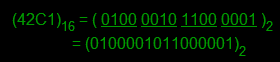Next generation website theme is here (black, neon and white color) - Reduces eye strain and headache problems.

## Hexadecimal Number System

Represents 16 types of digits from 0 to 9 and alphabets from A to F, so the base of number system is 16. Digits from 10 to 15 are represented as 10-A, 11-B, 12-C, 13-D, 14-E, 15-F. Conversion of this number system is also same as other numbers system.

### Hexadecimal to all conversion

Hexadecimal to binary conversion

Steps:

• Convert each hexadecimal digit into 4-bit binary equivalent.

Example:Hexadecimal to octal conversion

Steps:

• Converting each hexadecimal digit into 4-bit binary equivalent.
• Group these binary equivalent into 3-bit.
• Convert to octal digits.

Example:Hexadecimal to decimal conversion

Steps:

• Multiply each bit by 16n, where n is the weight of the bit.
• Weight is calculated by the position of bit, starting from 0 on the right.
• Add the results.

Example:## Tip Box

Hexadecimal number system is a human freindly representation of binary coded values in computing and digital electronics.